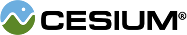#PrimitiveType

#### PrimitiveType() Core/PrimitiveType.js 15

The type of a geometric primitive, i.e., points, lines, and triangles.

### Members

#### staticconstantCesium.PrimitiveType.LINE_LOOP : Number Core/PrimitiveType.js 39

Line loop primitive where each vertex (or index) after the first connects a line to the previous vertex, and the last vertex implicitly connects to the first.

#### staticconstantCesium.PrimitiveType.LINE_STRIP : Number Core/PrimitiveType.js 47

Line strip primitive where each vertex (or index) after the first connects a line to the previous vertex.

#### staticconstantCesium.PrimitiveType.LINES : Number Core/PrimitiveType.js 30

Lines primitive where each two vertices (or indices) is a line segment. Line segments are not necessarily connected.

#### staticconstantCesium.PrimitiveType.POINTS : Number Core/PrimitiveType.js 22

Points primitive where each vertex (or index) is a separate point.

#### staticconstantCesium.PrimitiveType.TRIANGLE_FAN : Number Core/PrimitiveType.js 74

Triangle fan primitive where each vertex (or index) after the first two connect to the previous vertex and the first vertex forming a triangle. For example, this can be used to model a cone or circle.

#### staticconstantCesium.PrimitiveType.TRIANGLE_STRIP : Number Core/PrimitiveType.js 64

Triangle strip primitive where each vertex (or index) after the first two connect to the previous two vertices forming a triangle. For example, this can be used to model a wall.

#### staticconstantCesium.PrimitiveType.TRIANGLES : Number Core/PrimitiveType.js 55

Triangles primitive where each three vertices (or indices) is a triangle. Triangles do not necessarily share edges.